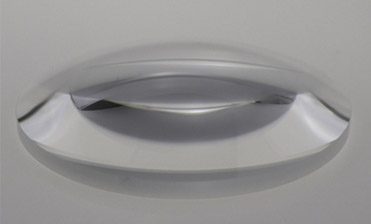# You need to know about the Lens

Oct. 29, 2019

Speaking of the convex mirror, in fact, it is a general term for a product. It is literally understood that the lenticular lens is divided into a concave lens and a convex lens.

Plano-Concave Lenses is also known as a negative-lens lens. The lens is thin in the middle and thick at the edges. It is also called a concave lens. The concave lens has a diverging effect on the light and can be used as a myopia mirror or an astigmatism mirror.Plano Convex Lenses

Plano Convex Lenses are based on the principle of light refraction. The convex lens is a lens with a thick center and a thinner edge.

The convex lens is divided into a form of double convex, flat-convex and concave (or positive meniscus). The convex lens has the function of collecting light, so it is also called a converging lens. The thick convex lens has the function of telephoto and convergence, which is related to the thickness of the lens. Can do magnifying glass, reading glasses.

What are the characteristics of the lenticular lens?

Features:

1. The reflection phenomenon on the lenticular mirror follows the law of reflection of light.

2. After the light parallel to the main axis is reflected by the concave mirror, the reflected light will converge at the focus. The focus of the lenticular lens is the convergence point of the actual light, so it is the real focus.

3. The lenticular lens converges on the light, so the smaller the focal length, the larger the convergence skill.

The concave lens imaging rule u is the object distance, v is the image distance, f is the focal length and is the same as the convex lens.

1. For thin concave lenses:

When an object is a real object, it becomes an erect, reduced virtual image and the object is on the same side of the lens;

When an object is a virtual object when the distance from the concave lens to the virtual object is within one focal length, it is an erect, magnified real image and the image is on the same side of the lens;

When an object is a virtual object, the distance from the concave lens to the virtual object is one focal length, and the image is at infinity;

When an object is a virtual object and the distance from the concave lens to the virtual object is less than twice the focal length of the focal length, the inverted, enlarged virtual image, the image is on the opposite side of the lens;

When an object is a virtual object, the distance from the concave lens to the virtual object is twice the focal length, and the virtual image is the same size as the object and the image is on the opposite side of the lens;

When an object is a virtual object and the distance from the concave lens to the imaginary object is less than twice the focal length, it is an inverted, reduced virtual image and the image is on the opposite side of the lens.

2. If it is a thick meniscus concave lens, the situation will be more complicated. When the thickness is large enough, it is equivalent to a Galileo telescope. When the thickness is larger, it is equivalent to a positive lens.

3. Our company is a Spherical Lenses Manufacturer. Welcome everyone to consult.